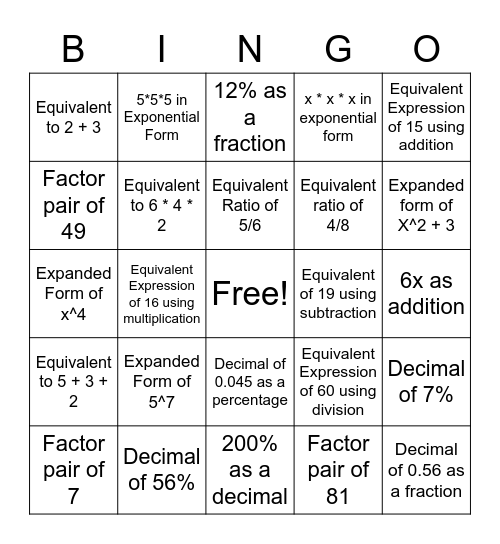# Equivalent ExpressionsThis bingo card has a free space and 24 words: Expanded Form of 5^7, Decimal of 56%, Equivalent Ratio of 5/6, Factor pair of 7, Equivalent Expression of 15 using addition, Equivalent to 2 + 3, Factor pair of 49, 5*5*5 in Exponential Form, Equivalent of 19 using subtraction, Decimal of 0.045 as a percentage, Equivalent ratio of 4/8, Expanded Form of x^4, Equivalent Expression of 60 using division, Decimal of 7%, Equivalent to 6 * 4 * 2, Factor pair of 81, 6x as addition, 12% as a fraction, Expanded form of X^2 + 3, Equivalent Expression of 16 using multiplication, Decimal of 0.56 as a fraction, Equivalent to 5 + 3 + 2, x * x * x in exponential form and 200% as a decimal.

## Play Online